# Spectral Leakage

September 15, 2012 · -

Have you ever analyzed(take FFT) a signal which has one or two main frequency components and found out that there are many more components than you expect even if there is no noise in the signal? One of the reasons why it has more frequency components is spectral leakage, which is mainly due to windowing.
Say, we have a discrete signal of $x[n]$,
FFT of a sequence $x[n]$

$X[k] = \sum_{n=0}^{N-1} x[n] e^{\frac{-j 2 \pi n k}{N}}$
where $w$ is sampled by $\frac{2\pi k}{N}$ for $k = 0,1, ..., N-1$.
FFT assumes the signal is periodic with period N and infinite duration. As the observation time of the signal is limited for a finite interval, infinite duration assumption cannot be satisfied. However, if the observation time is an integer multiple of the period of the signal, it would not cause a problem. This is because periodic signal and repeated signal results in the same signal.
On the other hand, if the observation time is not an integer multiple of the period, then frequency components of the signal change. There would be either discontinuties or overlaps in the signal when it is repeated over the observation time.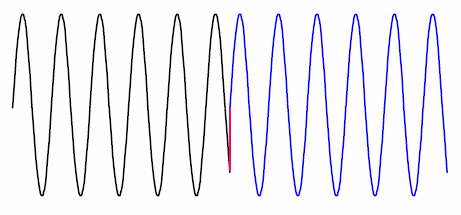The figure above shows the discontinuity in time domain and this results in spectral leakage in the frequency domain.
The problem is actually in this case and in general is “windowing”. FFT assumes the signal is periodic and infinite, but when we observe and actually get the signal, we get its some part in a window. This is called non-coherent sampling, and shown below also.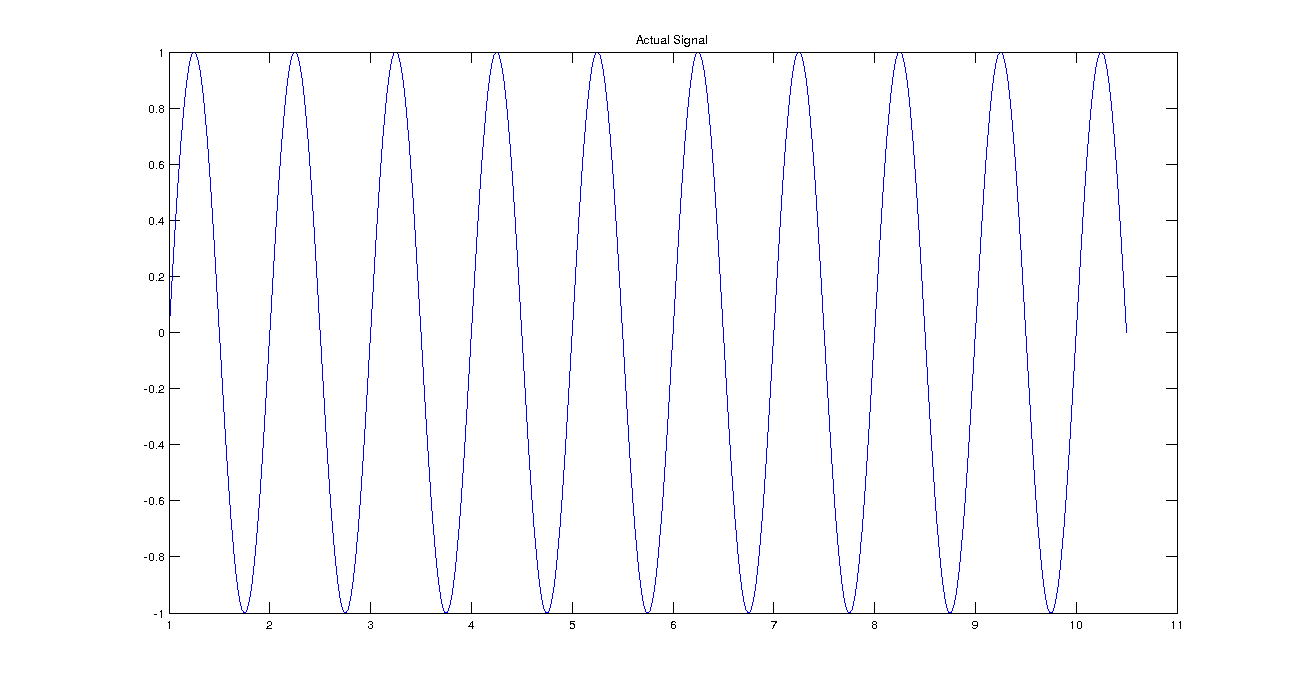That is the observed signal is actually convolution of “ideal”(infinite, periodic) signal with a windowing function. We also know that window results in $sinc$ function in the frequency domain and it has side lobes other than its main lobe. Those side lobes are not desired and they represent spectral leakage in frequency domain.
This results in unwanted frequency bins next to the main bin in the Fourier domain as shown below. It may be easy to remember this phenomena if you think a “leakage” from the main bin to the other bins.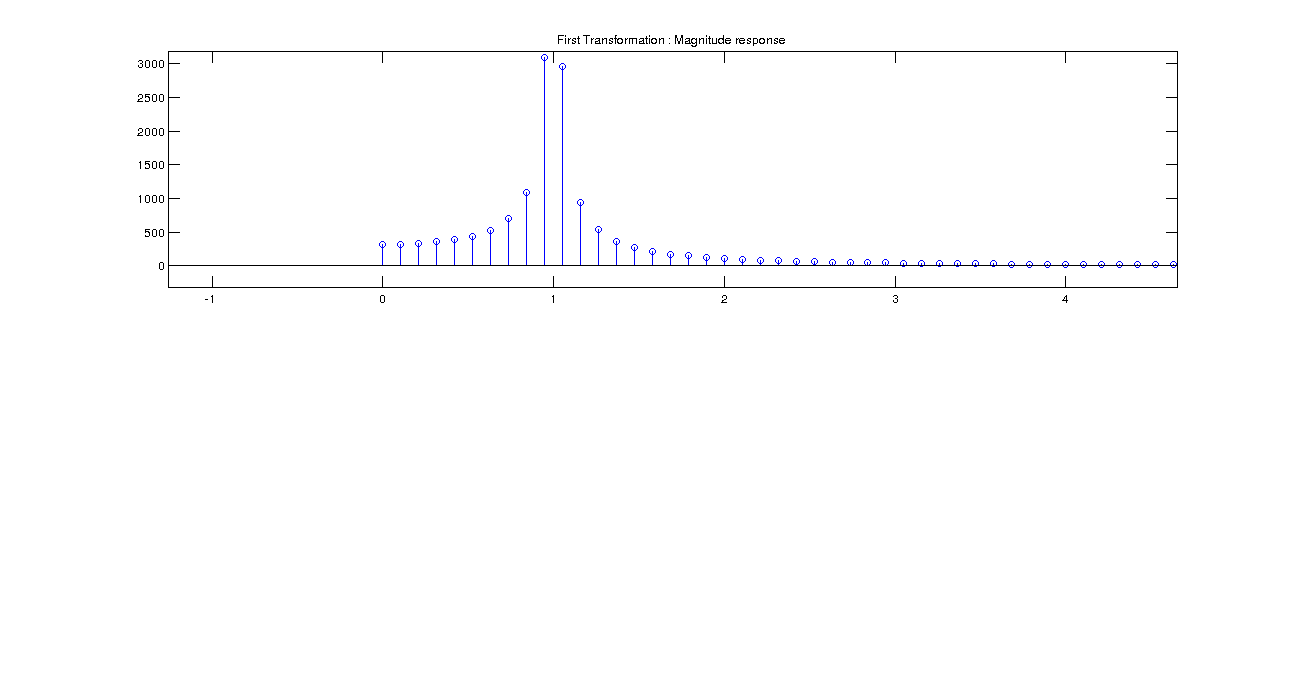If the sampling rate is the multiple integer of the period of the input signal, this sampling is called coherent sampling. However, it is not very common to know the input signal’s frequency exactly. Therefore, we need windows in order to analyze signals. Coherent sampling is shown in successive frames.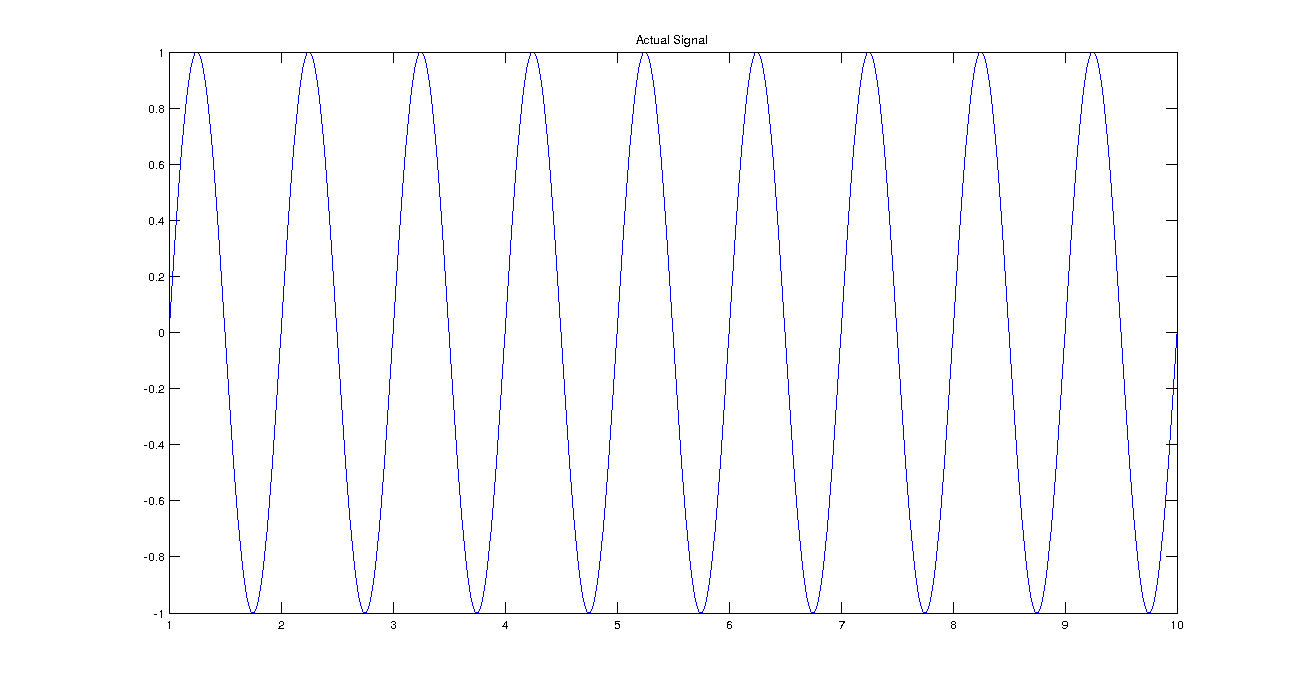Alt text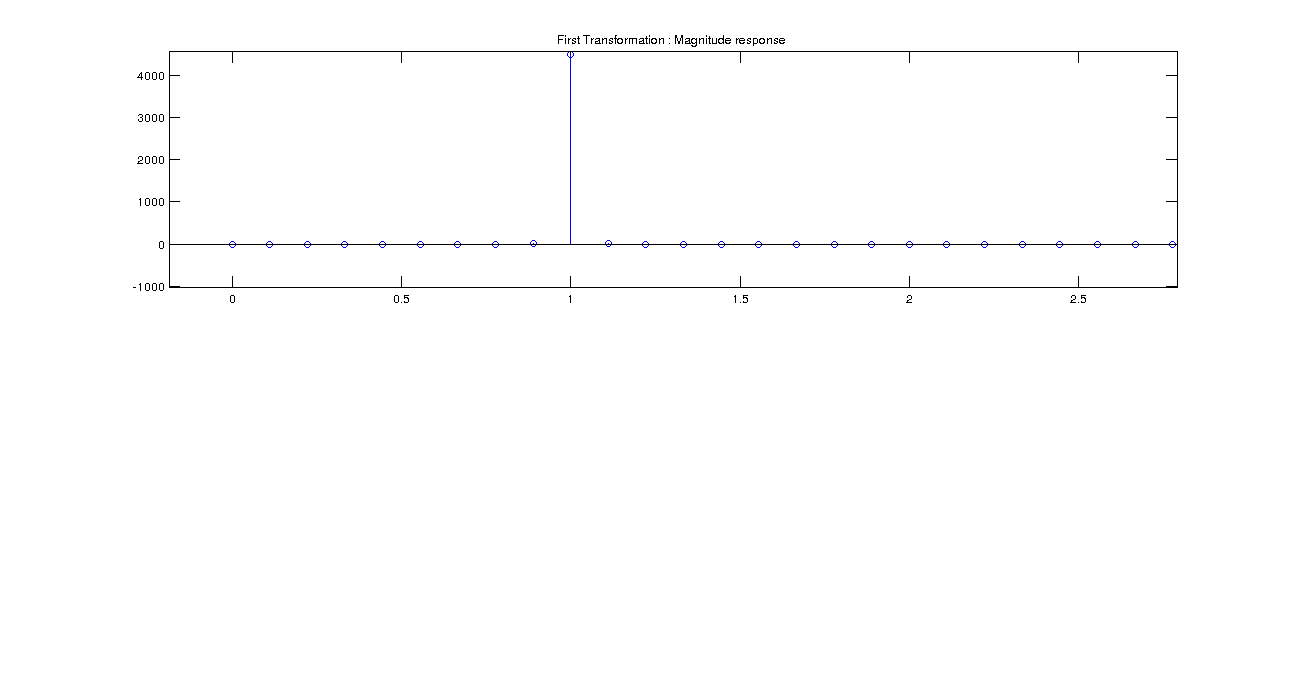Instead of rectangular signals, we may want to choose non-rectangular windows in order to decrease the spectral leakage. I do not want to go into detail, but in terms of choosing non-rectangular windows, there is also a trade-off. It is between frequency resolution and spectral leakage. If you want more frequency resolution in the signal, you need to sacrifice in the spectral leakage or vice versa.

### Code

All of the code and images used in this note is available in the here.

All Rights Reserved

Copyright, 2020